## Ep5 线性模型with Pytorch

news/2023/12/2 11:11:46

y=wx+b中w的扩充

# 4、torch.nn

（1）一般把网络中具有可学习参数的层（如全连接层、卷积层等）放在构造函数__init__()中，当然我也可以吧不具有参数的层也放在里面；

（2）一般把不具有可学习参数的层(如ReLU、dropout、BatchNormanation层)可放在构造函数中，也可不放在构造函数中，如果不放在构造函数__init__里面，则在forward方法里面可以使用nn.functional来代替.

（3）forward方法是必须要重写的，它是实现模型的功能，实现各个层之间的连接关系的核心。

Pytorch学习笔记07----nn.Module类与前向传播函数forward的理解 - 雨后观山色 - 博客园 (cnblogs.com)

# 6、三个魔法函数

python中所有对象都有一个从创建，被使用，再到消亡的过程，不同的阶段由不同的方法负责执行。

## 6.1 __new__()

`__new__` 方法的返回值就是类的实例对象，这个实例对象会传递给 `__init__` 方法中定义的 self 参数，以便实例对象可以被正确地初始化。

## 6.2 __int__()构造函数

Python入门 类class 基础篇 (zhihu.com)

``class Circle(object):  # 创建Circle类def __init__(self, r): # 初始化一个属性r（不要忘记self参数，他是类下面所有方法必须的参数）self.r = r  # 表示给我们将要创建的实例赋予属性r赋值``

self 代表类的实例，是通过类创建的实例

## 6.3  __call__()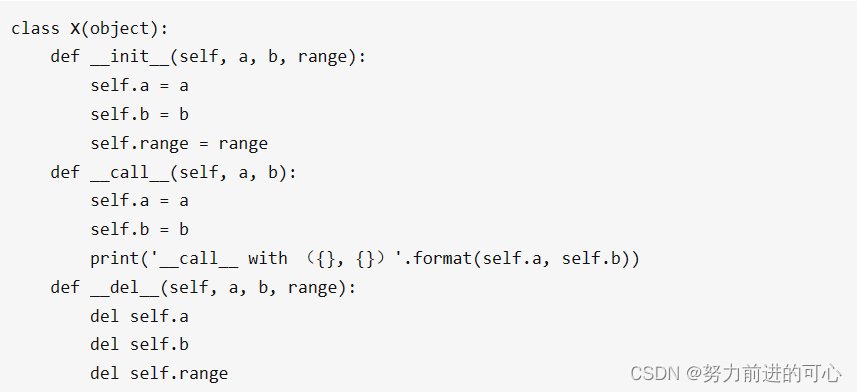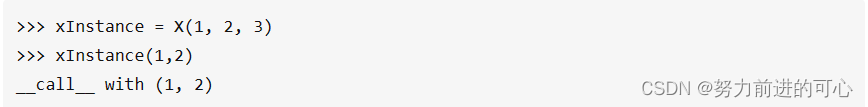1. xInstance = X(1, 2, 3) 这句代码实例化了xInstance类型的对象X，在实例化时调用了__init__(self, a, b, range)函数

2. xInstance(1,2)代码使用类+参数的语法 直接调用了 __call__(self, a, b)函数，而不需要使用X.__call__(self, a, b)语法，这就是__call__(self, a, b)函数的作用，能够让python中的类能够像方法一样被调用

#深入探究# PyTorch中的 forward() 方法详解 - 知乎 (zhihu.com)

# 7、如何定义类？

``````class people:#定义基本属性name = ''age = 0#定义私有属性,私有属性在类外部无法直接进行访问__weight = 0#定义构造方法def __init__(self,n,a,w):self.name = nself.age = aself.__weight = wdef speak(self):print("%s 说: 我 %d 岁。" %(self.name,self.age))# 实例化类
p = people('runoob',10,30)
p.speak()``````

(62条消息) python如何定义类？_python定义类_DongHappyyy的博客-CSDN博客

【python类包含方法】

self参数 说明：

【python类包含属性】

• 私有属性

函数、方法或者属性的名称以两个下划线开始，则为私有类型；

• 公有属性

如果函数、方法或者属性的名称没有以两个下划线开始，则为公有属性；

• 实例属性

以self作为前缀的属性；

• 局部变量

类的方法中定义的变量没有使用self作为前缀声明，则该变量为局部变量；

xxx 公有变量

_xxx "单下划线 " 开始的成员变量叫做保护变量，意思是只有类对象（即类实例）和子类对象自己能访问到这些变量，需通过类提供的接口进行访问；不能用'from module import *'导入

__xxx 类中的私有变量（Python的函数也是对象，所以成员方法称为成员变量也行得通。）," 双下划线 " 开始的是私有成员，意思是只有类对象自己能访问，连子类对象也不能访问到这个数据但可以通过私有属性，可以通过instance._classname_attribute方式访问

tip：python中有点伪私有属性的意思

Python 类>>>类属性（私有属性、公有属性、实例属性、局部变量）类方法（实例方法、静态方法） - 勿忘-前行 - 博客园 (cnblogs.com)

# 8、前向和后向

## 8.1 forward()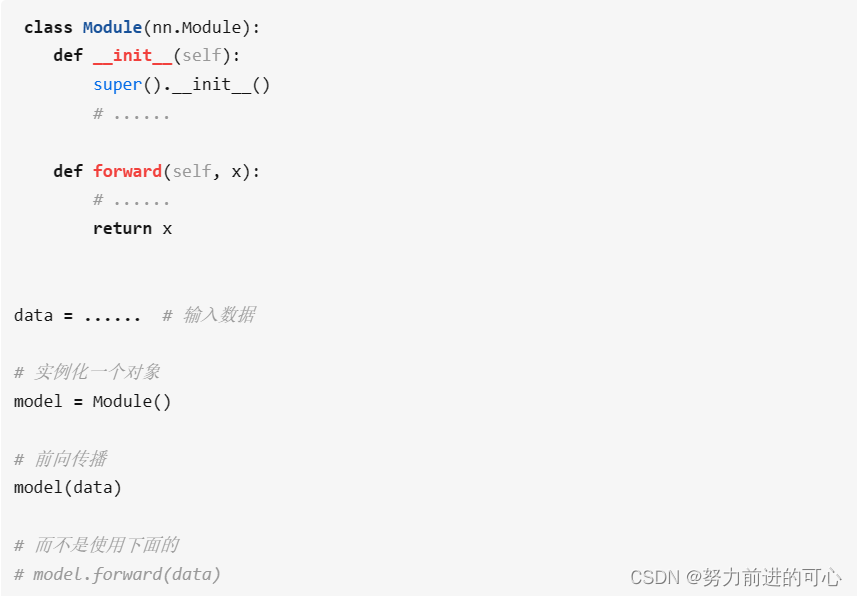## 8.2 backward()

loss.backward()故名思义，就是将损失loss 向输入侧进行反向传播，同时对于需要进行梯度计算的所有变量 x(requires_grad=True)，计算梯度 dloss/dx ，并将其累积到梯度x.grad中备用，即：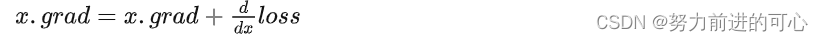在pytorch中，只有浮点类型的数才有梯度，因此在定义张量时一定要将类型指定为float型。

(62条消息) PyTorch：梯度计算之反向传播函数backward()_backward函数_精致的螺旋线的博客-CSDN博客

``````>>y = x + 2

x = torch.tensor([0.0, 2.0, 8.0], requires_grad=True)
y = torch.tensor([5.0, 1.0, 7.0], requires_grad=True)
z = x * y
print(z)

# (62条消息) PyTorch：梯度计算之反向传播函数backward()_backward函数_精致的螺旋线的博客-CSDN博客9. 类与子类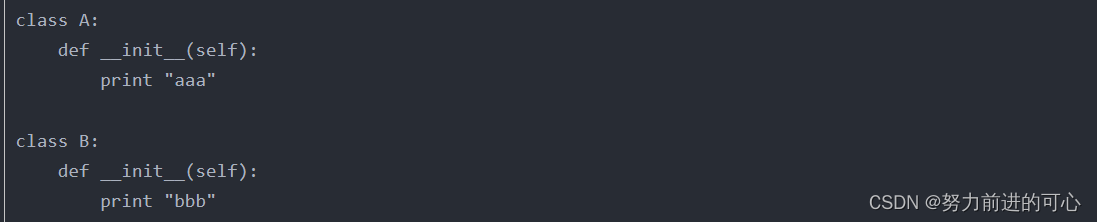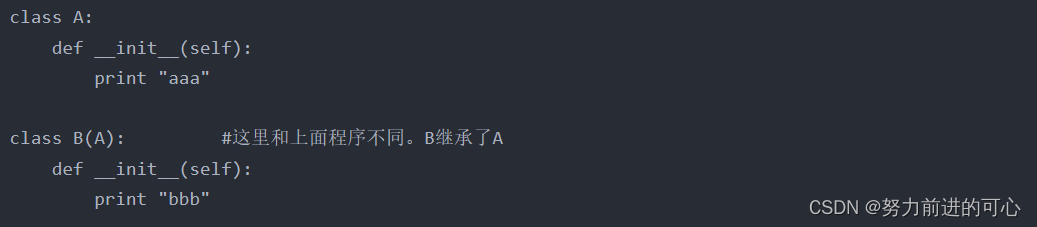B是A的子类

# 10、super().__inti__()

``````class A:def hi(self):print("A hi")class B(A):def hello(self):print("B hello")b = B()
b.hi()       # B里没有写hi(),这里调用的是继承自A的hi()------------------------------------------------------------------
class A:def hi(self):print("A hi")class B(A):def hi(self):print("B hi")b = B()
b.hi()    # 这里调用的就是B自己的hi()
------------------------------------------------------------------
class A:def hi(self):print("A hi")class B(A):def hi(self):super().hi()         # 通过super调用父类A的hi()print("B hi")b = B()
b.hi()    # 这里调用的就是A里面的hi()
``````

(62条消息) python中super().__init__()_BeanInJ的博客-CSDN博客

`torch.nn.Module` 这个类的内部有多达 48 个函数，这个类是 `PyTorch` 中所有 `neural network module` 的基类，自己创建的网络模型都是这个类的子类

# 作业

`optimizer = torch.optim.SGD(model.parameters(), lr=0.01) `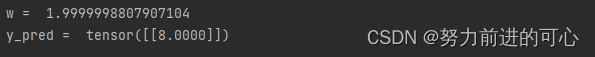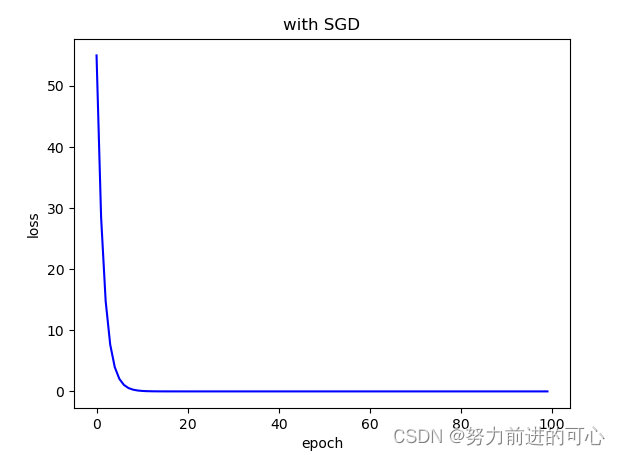`optimizer = torch.optim.Adam(model.parameters(), lr=0.1, betas=(0.9, 0.999), eps=1e-08, weight_decay=0)`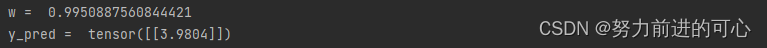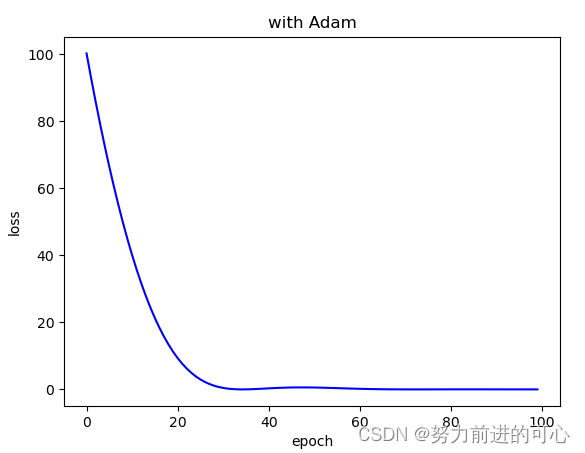`optimizer = torch.optim.ASGD(model.parameters(), lr=0.01, lambd=0.0001, alpha=0.75, t0=1000000.0, weight_decay=0)`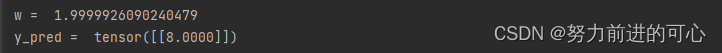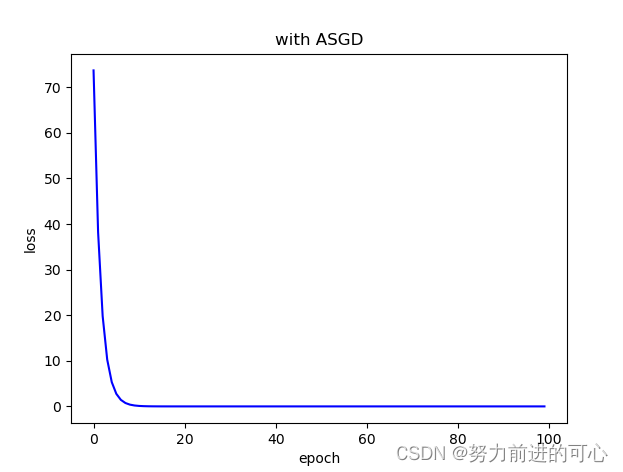代码：

``````import torch
import matplotlib.pyplot as plt# prepare dataset
# x,y是矩阵，3行1列 也就是说总共有3个数据，每个数据只有1个特征
x_data = torch.tensor([[1.0], [2.0], [3.0]])#创建Tensor
y_data = torch.tensor([[2.0], [4.0], [6.0]])# design model using class
"""
our model class should be inherit from nn.Module, which is base class for all neural network modules.
member methods __init__() and forward() have to be implemented
class nn.linear contain two member Tensors: weight and bias
class nn.Linear has implemented the magic method __call__(),which enable the instance of the class can
be called just like a function.Normally the forward() will be called
"""class LinearModel(torch.nn.Module):def __init__(self):super(LinearModel, self).__init__()##父类# (1,1)是指输入x和输出y的特征维度，这里数据集中的x和y的特征都是1维的# 该线性层需要学习的参数是w和b  获取w/b的方式分别是~linear.weight/linear.biasself.linear = torch.nn.Linear(1, 1, bias=False)def forward(self, x):y_pred = self.linear(x)return y_predmodel = LinearModel()
Loss_list = []
# construct loss and optimizer
# criterion = torch.nn.MSELoss(size_average = False)
criterion = torch.nn.MSELoss(reduction='sum')
optimizer = torch.optim.SGD(model.parameters(), lr=0.01)  #params:要训练的参数
#optimizer = torch.optim.Adam(model.parameters(), lr=0.1, betas=(0.9, 0.999), eps=1e-08, weight_decay=0)
# optimizer = torch.optim.ASGD(model.parameters(), lr=0.01, lambd=0.0001, alpha=0.75, t0=1000000.0, weight_decay=0)
# training cycle forward, backward, update
for epoch in range(100):y_pred = model(x_data)  # forward:predictloss = criterion(y_pred, y_data)  # forward: lossprint(epoch, loss.item())optimizer.zero_grad()  # the grad computer by .backward() will be accumulated. so before backward, remember set the grad to zeroloss.backward()  # backward: autograd，自动计算梯度optimizer.step()  # update 参数，即更新w和b的值Loss_list.append(loss)Loss_list_final = torch.tensor(Loss_list)
print('w = ', model.linear.weight.item())
#print('b = ', model.linear.bias.item())x_test = torch.tensor([[4.0]])
y_test = model(x_test)
print('y_pred = ', y_test.data)plt.figure()
plt.plot(Loss_list_final,'b',label = 'loss')
plt.ylabel('loss')
plt.xlabel('epoch')
plt.title('with SGD')
plt.show()``````

### HBase Shell 常用命令练习

HBase Shell 常用命令练习 前言一、HBase Shell是什么&#xff1f;二、HBase Shell使用步骤1.启动HBase2.启用HBase Shell3.键入HBase Shell命令操作HBase 三、常用HBase Shell实例1.常用的HBase Shell命令2.一个运用上述命令的综合实例&#xff1a; 总结 前言 提示&#xff1…

### 侃侃算法EP5·二叉树及其遍历

1. 前言 这个板块旨在记录一些日常中或是面试中会问到的算法和数据结构相关的内容&#xff0c;更多是给自己总结和需要的人分享。在内容部分可能由于我的阅历和实战经历不足&#xff0c;会有忽视或是写错的点&#xff0c;还望轻喷。 2. 内容 关于什么是树、子树、根节点、叶…

### JS高级+ES678

js高级 数据类型 基本(值)类型 Number: 任意数值String: 任意文本Boolean: true/falseundefined: undefinednull: null 对象(引用)类型 Object: 任意对象 主要用来包含无序复杂的数据Array: 特别的对象类型(下标/内部数据有序)Function: 特别的对象类型(可执行)&#xff0c;F…

### Elasticsearch8.0

Elastic 中国社区官方博客_CSDN博客-Elastic,Elasticsearch,Kibana领域博主Elastic 中国社区官方博客擅长Elastic,Elasticsearch,Kibana,等方面的知识https://elasticstack.blog.csdn.net/ ✅ 启动 elasticsearch # cd /usr/local/elastic/elasticsearch/ # ./bin/elasticsearc…

### ES6/ES7/ES8/ES9/ES10

ES10 ES10 功能完全指南 好犯困啊 我来打打字 string.prototype.matchAll() ‘Hello’.match(‘l’) eg:判断字符串中存在几个某元素 yannanna’.match(/n/g).length 扁平化多维数组&#xff08;想不出啥时候会用到&#xff09; let array [1, 2, 3, 4, 5]; array.map(x &g…

### es 客户端

ES客户端&#xff1a;Elasticsearch Clients 语言无关性 Java REST ClientJava APIPython APIGo API.Net APIPHP APIJavaScripts APIRuby APIPerl APIElandRustCommunity Contributed Clients Java API 生命周期&#xff08;生卒年&#xff1a;ES 0.9 - ES 7.x&#xff09;…

### ES7，ES8，ES10新特性

ES7 ES7在ES6的基础上增加了三项内容 求幂运算符 ** console.log(3 ** 2 ) // 9 Array.prototype.includes()方法 includes()的作用是查找一个值在不在数组中&#xff0c;接受两个参数&#xff1a;搜索值和搜索的开始索引。如果没有传递参数默认的索引是0 // 下面的这两种方…

### ES7、ES8、ES9、ES10新特性

ES7新特性 1.Array.prototype.includes()方法 在ES6中我们有String.prototype.includes()可以查询给定字符串是否包含一个字符，而在 ES7 中，我们在数组中也可以用 Array.prototype.includes 方法来判断一个数组是否包含一个指定的值，根据情况，如果包含则返回true，否则返…